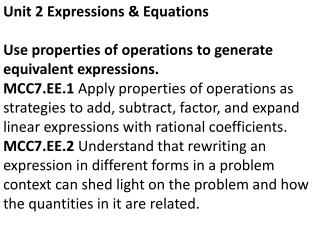DownloadDownload PresentationUnit 2 Expressions & Equations

# Unit 2 Expressions & Equations

Télécharger la présentation## Unit 2 Expressions & Equations

- - - - - - - - - - - - - - - - - - - - - - - - - - - E N D - - - - - - - - - - - - - - - - - - - - - - - - - - -
##### Presentation Transcript

1. Unit 2 Expressions & Equations Use properties of operations to generate equivalent expressions. MCC7.EE.1 Apply properties of operations as strategies to add, subtract, factor, and expand linear expressions with rational coefficients. MCC7.EE.2 Understand that rewriting an expression in different forms in a problem context can shed light on the problem and how the quantities in it are related.

2. Draw a number line like the one above and model each problem. (-2) + (-6) = 5 + ( -6) =